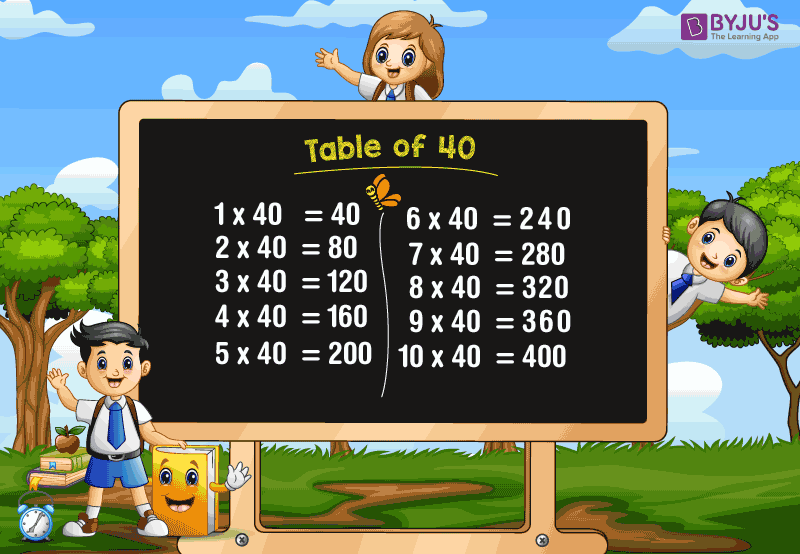# Table of 40

Table of 40 is given here to help the students, and it is extremely useful, as direct questions are often asked from the multiples of 40 in school exams as well as in the competitive exams. The table of 40 can be easily learned if you know the table of 4. The multiplication table of 40 is provided in the tabular form, and also learn how to read the forty times table in this article.

### Download Table of 40 Here## 40 Times Table

If you know the table of 4, you can easily learn the table of 40. For example, 4 times 5 is 20. Similarly, 40 times 5 is 200. It means that you just add “0” at the end of each answer. The multiplication table of 40 gives the multiples of 40, which is nothing but the multiplication of the number 40 with different whole numbers. Similarly, if you add the number 40 repeatedly for the number of times, you will get the table of 40.

For example, 40 times 3 is 120.

(i.e) 40 × 3 =120.

The same result will be obtained by adding the number 40 for 3 times.

(i.e.) 40+40+40 =120

Also, check tables from 1 to 100 for more details.

## Multiplication Table of 40

The table of 40 is provided in tabular form to solve the problems quickly. The multiplication of 40 with numbers from 1 to 20 are given below:

 40 × 1 = 40 40 × 2 = 80 40 × 3 = 120 40 × 4 = 160 40 × 5 = 200 40 × 6 = 240 40 × 7 = 280 40 × 8 = 320 40 × 9 = 360 40 × 10 = 400 40 × 11 = 440 40 × 12 = 480 40 × 13 = 520 40 × 14 = 560 40 × 15 = 600 40 × 16 = 640 40 × 17 = 680 40 × 18 = 720 40 × 19 = 760 40 × 20 = 800

### How to Read 40 Times Table?

Read the table of 40 as follows:

• One time forty is 40
• Two times forty is 80
• Three times forty is 120
• Four times forty is 160
• Five times forty is 200
• Six times forty is 240
• Seven times forty is 280
• Eight times forty is 320
• Nine times forty is 360
• Ten times forty is 400
• Eleven times forty is 440
• Twelve times forty is 480
• Thirteen times forty is 520
• Fourteen times forty is 560
• Fifteen times forty is 600.

Get More Maths Tables:

## Frequently Asked Questions on Table of 40

### 1. What is the table of 40?

The multiplication table of 40 gives the multiples of 40. The multiplication of number 40 with different numbers results in the 40 times table.

### 2. What is the value of 15 times 40?

15 times 40 is 600. (i.e.) 15 × 40 = 600.

### 3. What are the first 10 multiples of 40?

The first ten multiples of 40 are 40, 80, 120, 160, 200, 240, 280, 320, 360, and 400.

### 4. What is the cost of 7 books, if each book costs Rs. 40?

The cost of one book = Rs. 40
Then, the cost of 7 books = 7×40 = 280
Hence, the cost of 7 books is Rs. 280.

### 5. Find the value of x, if 40x=360.

The value of x is 9.
Explanation: 40x= 360
x = 360/40
x =9.